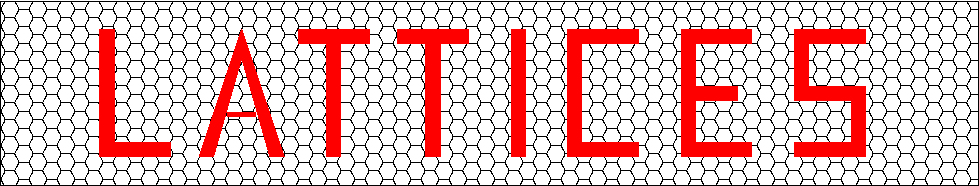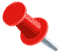## Modular Lattices (Footnotes to Table)Keywords: modular lattices, tables, minimal norm, quadratic formsPart of the Catalogue of Lattices which is a joint project of Gabriele Nebe, RWTH Aachen university (nebe@math.rwth-aachen.de) and Neil J. A. Sloane, (njasloane@gmail.com).## Footnotes to Table of Modular Lattices

### Constructions

(d) Direct sum: If L and M are N-modular so is the direct sum L + M. If L has genus l (= +-1) and M has genus m (= +-1) then L + M has genus lm.

(B) Constructed by C. Bachoc: see Reference [Bach97] below.

(C) Construction A applied to an additive trace self-dual code of length n and min distance d over GF(4) produces a 3-modular lattice in dim 2n and minimal norm = min{d, 4} - see References [Bach97], [RaSl98] below.

(Q) Constructed (or mentioned) by H.-G. Quebbemann: see References [Queb95], [Queb97] below.

### Upper Bounds

(g) By a complete investigation of the genus, R. Scharlau and B. Hemkemeier [SchHe94] show that no even lattice exists. From [RaSl98], an extremal lattice would necessarily be even. So minimal norm 6 is impossible.

(S) Shadow plus integrality: The minimal norm cannot be greater than shown, or else one of the following conditions fails: theta functions of lattice and shadow have nonnegative integer coefficients, theta function of lattice is congruent to 1 mod 2, theta function of shadow is congruent to 0 or 1 mod 2; shadow may contain at most two vectors of norm less than half the minimal norm of the lattice.

### References

• [Bach97] C. Bachoc, Applications of coding theory to the construction of modular lattices, J. Combin. Theory, A 78 (1997), 92-119.
• [Queb95] H.-G. Quebbemann, Modular lattices in Euclidean spaces, J. Number Theory 54 (1995), 190-202.
• [Queb97] H.-G. Quebbemann, Atkin-Lehner eigenforms and strongly modular lattices, L'Enseign. Math. 43 (1997), 55-65.
• [RaSl98] E. M. Rains and N. J. A. Sloane, The shadow theory of modular and unimodular lattices, J. Number Theory, 73 (1998), 359-389.
• [SchHe94] R. Scharlau and B. Hemkemeier, Classification of integral lattices with large class number, Preprint No. 94-102, Univ. Bielefeld, 1994.
• [SchVe95] R Scharlau and B. B. Venkov, The genus of the Barnes-Wall lattice, Comm. Math. Helv., 69 (1994), 322-333.